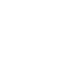# ACT & SAT Question of the Day: Apr. 10, 2014

If you are reading this in an email you received from me, do not click the link to sat.collegeboard.org below. Use the link to my website that is farther down on the email.

http://sat.collegeboard.org/practice/sat-question-of-the-day?questionId=20140410&oq=1 (This link takes you to today’s question. If you use my archive, you will see the question related to my SAT explanation for that date.)

The answer is A.  This question isn’t very hard but only 70% of students are getting it correct.  Let’s do it and then consider the larger issue of taking the test.   Change what the test writer gives you to an inequality: p x 75 > 75.  For that to be true, p has to be greater than 1.  That means the answer has to be A.

Let’s think about this question.  What can we learn about the test from it?  I find that a surprising number of students miss percent questions.  Sometimes there are percent questions late in the test (meaning that well over half of the students miss them) but the computation required is quite simple.  Why are percent questions harder than you might expect?  Let me warn you about a few things.

First, it has been a long time since you had to do percent questions; so, I’m guessing your skills are a little rusty.  Use the “Percent” lesson in my student manual, website, or videos to remind you how to set up the calculations that are required by the different kinds of percent questions you will see.

Second, sometimes the wording throws students off.  For example, students often miss percent questions because they confuse “of” and “more” or “less” than.  Remember that 75% “of” a number is 25% “less than” that number!  If you get confused, think about a dollar and what you have to do with it to answer the question.  (75% of a dollar is 75 cents and 25% less than a dollar is also 75 cents.)  Then apply the calculation you use for a dollar to the question in the test booklet.  (“Of” means multiply.  “Less than” means subtract.  “More than” means add.)

Third, remember that you can’t add two-step percentages; you must multiply them.  For example, if you skip 10% of the reading questions on the SAT and you miss 10% of the questions you do, you get 81% of the 67 SAT reading questions correct, not 80%!  (“Of” means multiply.  .90 times .90 is .81.)

The bottom line is you need to practice and all of this will come back to you.  You are probably going to see 1 or 2 percent questions on the test.  Be sure to do some percent questions and let me know if you have trouble.  I’ll help you out.

Let’s see what the ACT folks have for us today.

We just saw this question less than a week ago and I explained it in detail then.  So, if you weren’t reading my blog last week, here’s a short explanation.

The answer is A.  The only place on the table that is the same for both depths is for O2.  The ACT Science is about reading data displays quickly.  It isn’t about reviewing science information before the test.

QotD Words of “Wiz-dom”:

The ACT is coming up the day after tomorrow.  Read my blog archive about what to eat for breakfast on test day.  It could make a difference in your score.  Here it is: Breakfast Before the Test.  Be sure to tune back in tomorrow and I’ll remind you what to do tomorrow night before you take the test the next morning.

Have a great day!

Bob Alexander, the “SAT and ACT Wizard”